# Bases

The length of the bases trapezium are in ratio 4:5. Length of midline is 15. How long are the bases of a trapezoid?

x =  13.33
y =  16.67

### Step-by-step explanation:

(x+y)/2 = 15; 5 x = 4 y

(x+y)/2 = 15
5•x = 4•y

x+y = 30
5x-4y = 0

x = 40/3 ≈ 13.333333
y = 50/3 ≈ 16.666667

Our linear equations calculator calculates it.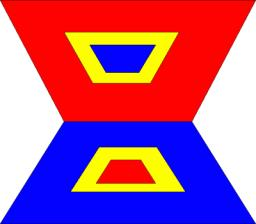Did you find an error or inaccuracy? Feel free to write us. Thank you!Tips to related online calculators
Looking for help with calculating arithmetic mean?
Looking for a statistical calculator?
Do you have a linear equation or system of equations and looking for its solution? Or do you have a quadratic equation?
Do you want to convert length units?

#### You need to know the following knowledge to solve this word math problem:

We encourage you to watch this tutorial video on this math problem:

## Related math problems and questions:

• Five numbers in ratioFive integers are in the ratio 1: 2: 3: 4: 5. Their arithmetic mean is 12. Determine the smallest of these numbers.
• Freight train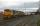The train carries 525 tons of limestone in 29 wagons. Wagons are 15 tonne and 20 tons. How many is 15 ton and how many is 20 ton wagons?
• Isosceles trapezoidCalculate the content of an isosceles trapezoid whose bases are at ratio 5:3, the arm is 6cm long and it is 4cm high.
• Trousers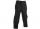Jarek bought new trousers, but the trousers were too long. Their length was in the ratio 5: 8 to Jarek height. Mother his trousers cut by 4 cm, thus the original ratio decreased by 4%. Determine Jarek's high.
• The rectangularThe rectangular trapezoid has bases 15 dm and 8 dm long and the length of the inclined arm is 12 dm. How long is the other arm of the trapezoid?
• Meanif the mean of the set of data 5, 17, 19, 14, 15, 17, 7, 11, 16, 19, 5, 5, 10, 8, 13, 14, 4, 2, 17, 11, x is -91.74, what is the value of x?
• Railway embankmentThe railway embankment section is an isosceles trapezoid, the sizes of the bases of which are in the ratio 5: 3. The arms have a length of 5 m, and the height of the embankment is 4.8 m. Calculates the size of the embankment section area.
• Speed of Slovakian trainsRudolf decided to take the train from the station 'Ostratice' to 'Horné Ozorovce'. In the train timetables found train Os 5409 : km 0 Chynorany 15:17 5 Ostratice 15:23 15:23 8 Rybany 15:27 15:27 10 Dolné Naštice 15:31 15:31 14 Bánovce nad Bebravou 15:35 1
• MG=7x-15,Length of lines MG = 7x-15 and FG = 33 Point M is the midpoint of FG. Find the unknown x.
• Trapezoid ABCD v2Trapezoid ABCD has a length of bases in ratio 3:10. The area of triangle ACD is 825 dm2. What is the area of trapezoid ABCD?
• Two numbers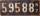Determine the numbers x and y so x + y = 8 is truth and the numbers are in the ratio of 4: 5.
• Tributaries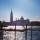The first tributary fill pool with water in 15 hours. The second tributary fill pool in 10 hours. For how many hours the pool is filled with both tributaries?
• Isosceles triangle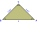In an isosceles triangle, the arm's length and the length of the base are in ratio 3 to 5. What is the length of the arm?
• Isosceles trapezoidThe lengths of the bases of the isosceles trapezoid are in the ratio 5:3, the arms have a length of 5 cm and height = 4.8 cm. Calculate the circumference and area of a trapezoid.
• Trapezoid MO-5-Z8Trapezoid KLMN has bases 12 and 4 cm long. The area of triangle KMN is 9 cm2. What is the area of the trapezoid KLMN?
• Classs meanClass size is 30 students, math was not worse than sign 2. Determine the number of pupils who were sign 1 if the class had a 1.4 sign average.
• Waiting roomIn the waiting room are people and flies. Together they have 15 heads and 50 legs (fly has 6 legs). How many people and flies are in the waiting room?